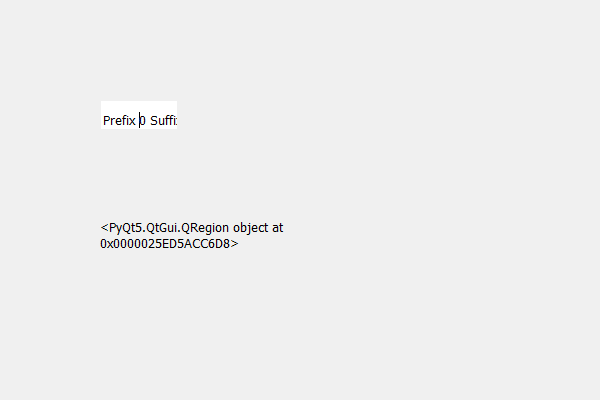# PyQt5 QSpinBox – Getting the Mask

In this article we will see how we can get the mask of the spin box, mask are basically used to hide the spin box, with the help of mask user will not be able to see the whole spin box although the spin box will still exist but there will be mask on it. Mask can be set to the spin box with the help of `setMask` method.

In order to get the mask we use `mask` method.

Argument : It takes no argument

Return : It returns QRegion object

Below is the implementation

 `# importing libraries ` `from` `PyQt5.QtWidgets ``import` `*`  `from` `PyQt5 ``import` `QtCore, QtGui ` `from` `PyQt5.QtGui ``import` `*`  `from` `PyQt5.QtCore ``import` `*`  `import` `sys ` ` `  ` `  `class` `Window(QMainWindow): ` ` `  `    ``def` `__init__(``self``): ` `        ``super``().__init__() ` ` `  `        ``# setting title ` `        ``self``.setWindowTitle(``"Python "``) ` ` `  `        ``# setting geometry ` `        ``self``.setGeometry(``100``, ``100``, ``600``, ``400``) ` ` `  `        ``# calling method ` `        ``self``.UiComponents() ` ` `  `        ``# showing all the widgets ` `        ``self``.show() ` ` `  `        ``# method for widgets ` `    ``def` `UiComponents(``self``): ` `        ``# creating spin box ` `        ``self``.spin ``=` `QSpinBox(``self``) ` ` `  `        ``# setting geometry to spin box ` `        ``self``.spin.setGeometry(``100``, ``100``, ``250``, ``40``) ` ` `  `        ``# setting range to the spin box ` `        ``self``.spin.setRange(``0``, ``999999``) ` ` `  `        ``# setting prefix to spin ` `        ``self``.spin.setPrefix(``"Prefix "``) ` ` `  `        ``# setting suffix to spin ` `        ``self``.spin.setSuffix(``" Suffix"``) ` ` `  `        ``# getting the region of children of spin box ` `        ``region ``=` `self``.spin.childrenRegion() ` ` `  `        ``# setting mask ` `        ``self``.spin.setMask(region) ` ` `  `        ``# creating label ` `        ``label ``=` `QLabel(``self``) ` ` `  `        ``# setting geometry to the label ` `        ``label.setGeometry(``100``, ``200``, ``200``, ``70``) ` ` `  `        ``# making it multi line label ` `        ``label.setWordWrap(``True``) ` ` `  `        ``# getting mask ` `        ``mask ``=` `self``.spin.mask() ` ` `  `        ``# setting to the label ` `        ``label.setText(``str``(mask)) ` ` `  `# create pyqt5 app ` `App ``=` `QApplication(sys.argv) ` ` `  `# create the instance of our Window ` `window ``=` `Window() ` `window.spin.setFocus() ` `# start the app ` `sys.exit(App.``exec``()) `

Output :Whether you're preparing for your first job interview or aiming to upskill in this ever-evolving tech landscape, GeeksforGeeks Courses are your key to success. We provide top-quality content at affordable prices, all geared towards accelerating your growth in a time-bound manner. Join the millions we've already empowered, and we're here to do the same for you. Don't miss out - check it out now!

Previous
Next